Have questions? Need Help?
757.723.6531 | 800.950.2468

# Teledyne Hastings Instruments Blog

Using thermal mass flow instruments by Teledyne Hastings is an easy way to quickly and accurately measure gas flow. And in some cases, a mass flow instrument may be calibrated for one gas, but then the user may want to use the instrument in another gas. In this blog, we will show how to use GCFs (Gas Conversion Factors) when using flow instruments in different gases.

Before we get into GCFs, let’s quickly review the operation of one of our flow sensors. Below, we show a diagram of the 200 Series flow sensor. In this sensor, gas flows through a capillary tube which is heated in the middle to a temperature which is approximately 130°C. Two thermocouples, one upstream (TC-1) and one downstream (TC-2), measure the temperature. The temperature difference between the two thermocouples is proportional to the heat flow through the capillary tube. The heat flow, in turn, is proportional to the mass flow times the specific heat Cp of the gas. So, to first order, if we want to use a thermal mass flow meter that has been set up for one gas, and use it with another gas, we will multiply the output of the meter by the ratio of the specific heats. GCF ~ Cp1 / Cp2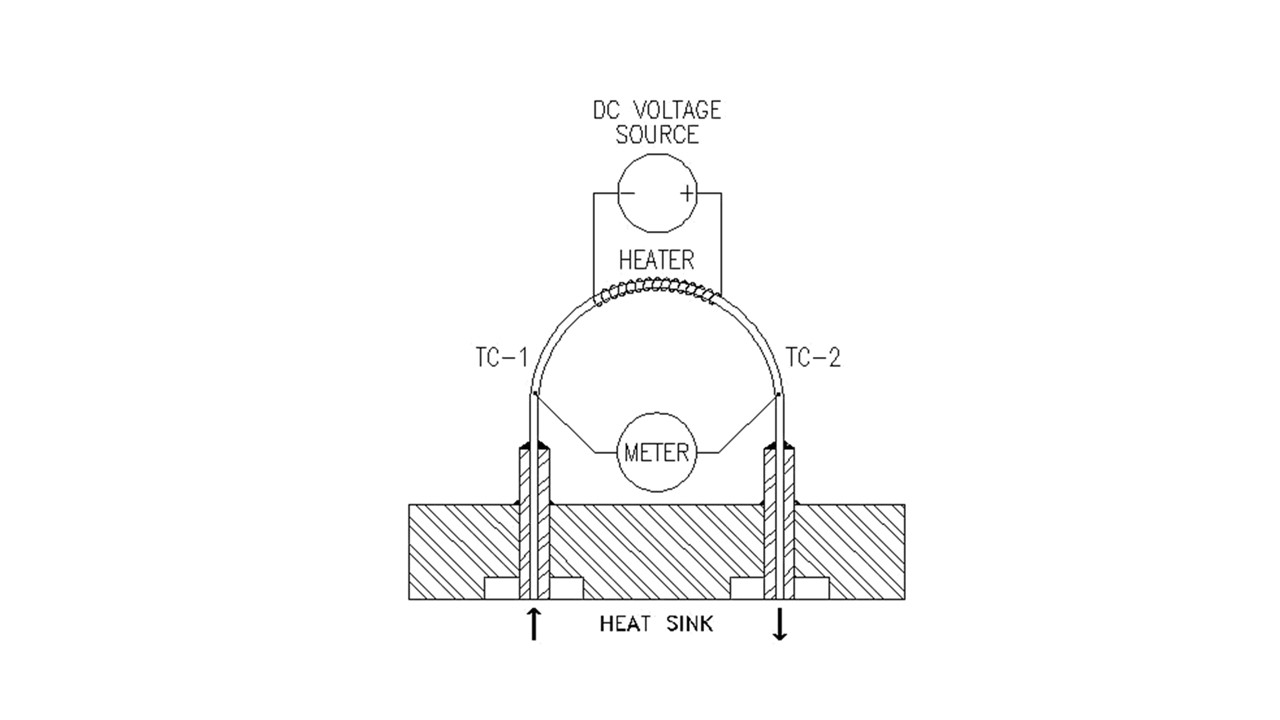There are a couple of things we need to point out. First, the ratio shown above is a simple approximation and does not tell the whole story. Next, the best GCFs are those that have been measured experimentally. However, in the case of dangerous gases, we use the best thermodynamic data available.

Here is a table of some common GCFs.

 Gas Conversion Factors (N2) 200 Series 300 Series Helium 1.402 1.400 Oxygen 0.981 0.978 Carbon Dioxide 0.743 0.753 Carbon Monoxide 1.001 1.001 Methane 0.770 0.779 Ammonia 0.781 0.781 Hydrogen 1.009 1.004 Argon 1.401 1.405

Next, we will discuss how we apply GCFs in practice. Let’s take an example of a flow meter that is calibrated for nitrogen. If we wanted to use the flowmeter in argon, we would take the output and multiply by the GCF for Argon.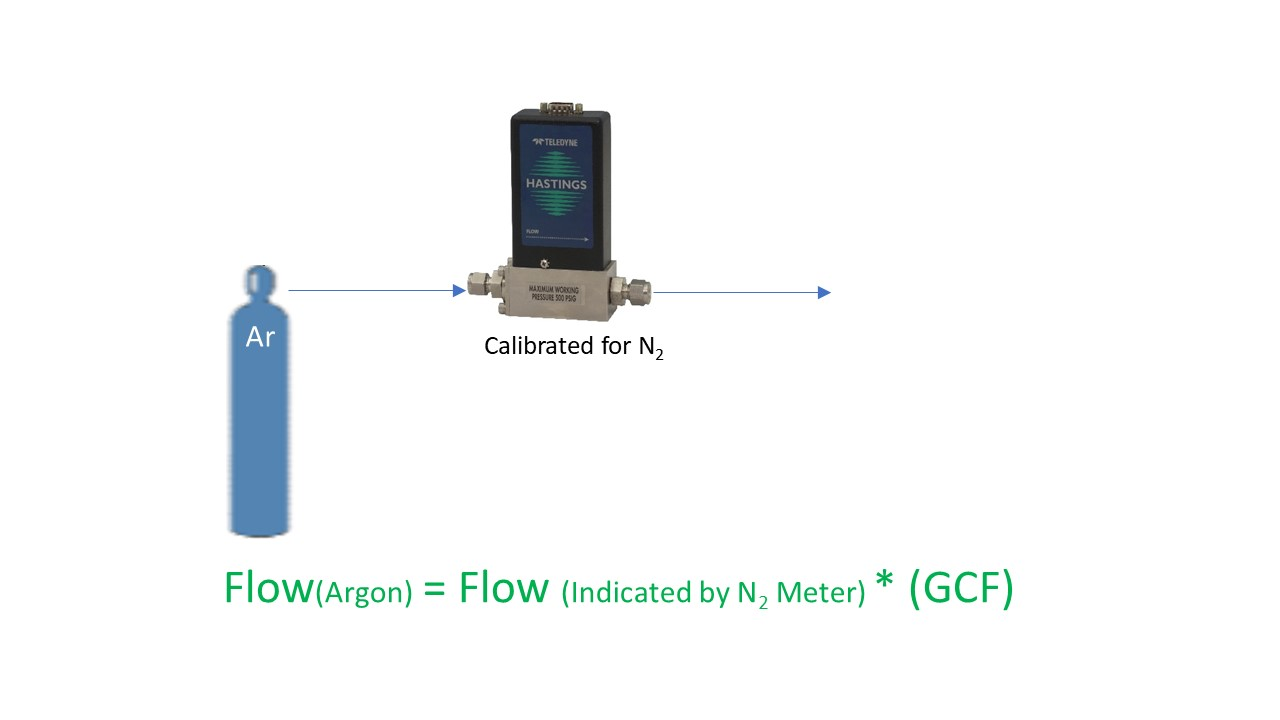Here is another example; suppose we have a meter that is calibrated in helium and we want to use it in hydrogen. You would start by dividing the output by the GCF for helium (think of it as converting to the nitrogen equivalent), and then multiplying by the GCF for hydrogen.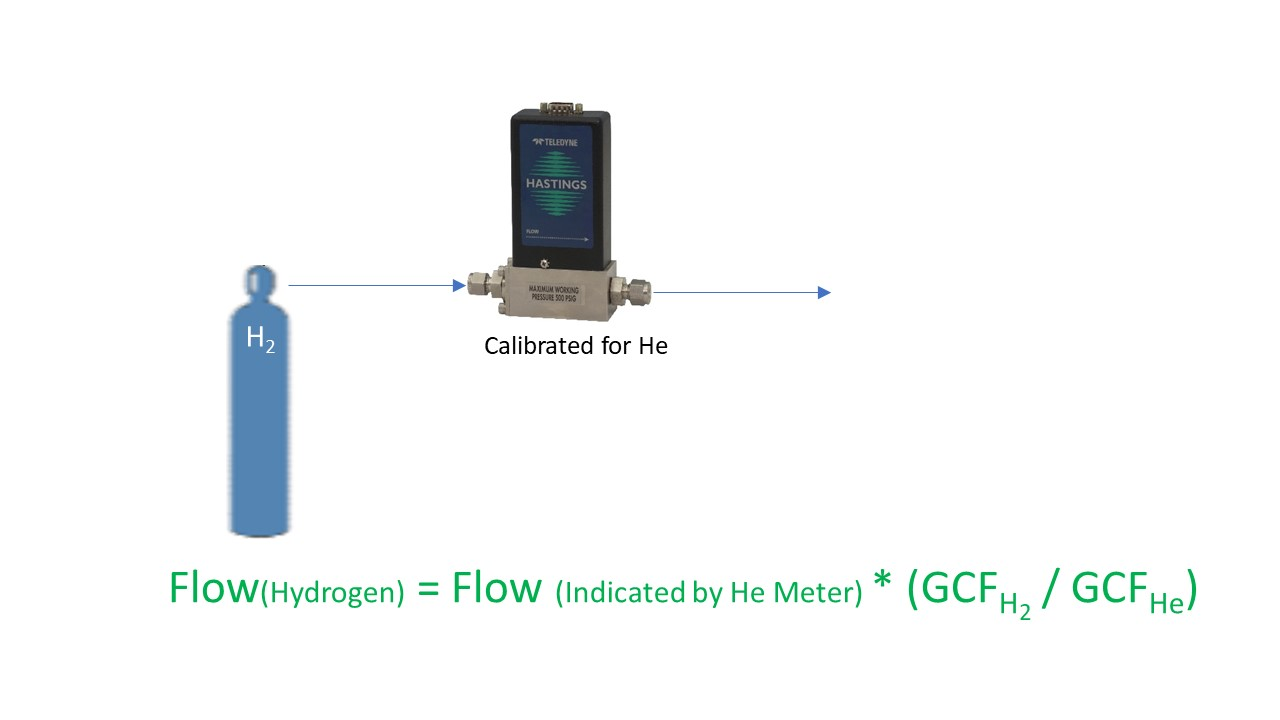Remember, always use the appropriate set of GCFs for the flow series that you are using. In other words, if you are using our Digital 300 Series, don’t apply GCFs from a 200 Series manual – they are not the same. And certainly don’t use non-Teledyne table of GCFs for use with Teledyne flow products. They might get you in the ballpark, but they will not be your best conversion.

One other quick note about applying GCFs. Our line of flow power supplies, the THCD-101 (single channel) and the THCD-401 (four channel), can be used to quickly scale the analog input which is equivalent to applying a conversion factor. Let’s take another look at the Argon example. If we used the THCD-101 power supply with the nitrogen flow meter as shown below, at the nominal full scale of the flow meter, we will have a 5 VDC signal. If we want to use this same meter and power supply with Argon, we just need to “tell” the THCD-101 what value to display when it receives 5 VDC. So, if our flow meter was calibrated for nitrogen to give 5 VDC at 250 sccm, then the same flow meter will give 5 VDC in argon at 350 sccm. (250 * 1.4 = 350). So, we would then range the THCD-101 for 350 sccm. This can be done from the front panel or via the internal webserver.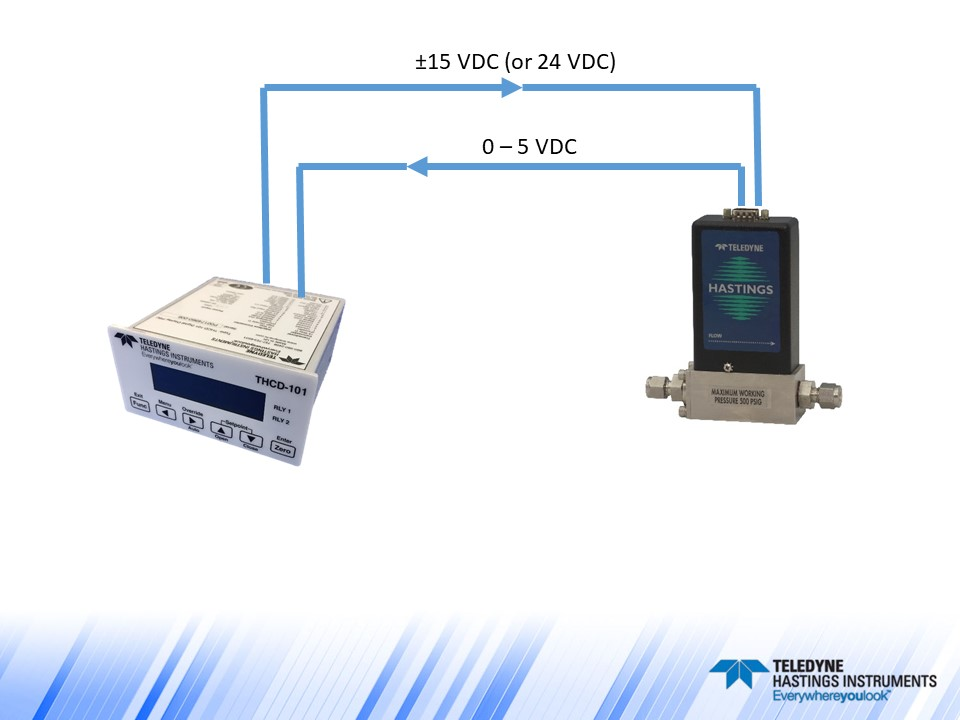Now let’s make things a little more interesting and discuss a flow controller example. Analog flow controllers work by receiving a command signal (usually 0-5 VDC, or 4-20 mA) and then they adjust their control valve such that the flow, and thus the analog signal output, matches the command signal input. (You can think of it like the cruise control in your car – you tell it you want to go 78 miles per hour, and then the engine does what it needs to do to maintain that speed). In the case of a 0-5 VDC flow controller, a 5-volt setpoint command is instructing the flow controller to set the flow to 100% of full scale. The relationship between flow rate and command signal is linear, so if the user wanted to control at 25% of full scale, then they would send a 1.25 VDC command signal (0.25 * 5 VDC = 1.25 VDC).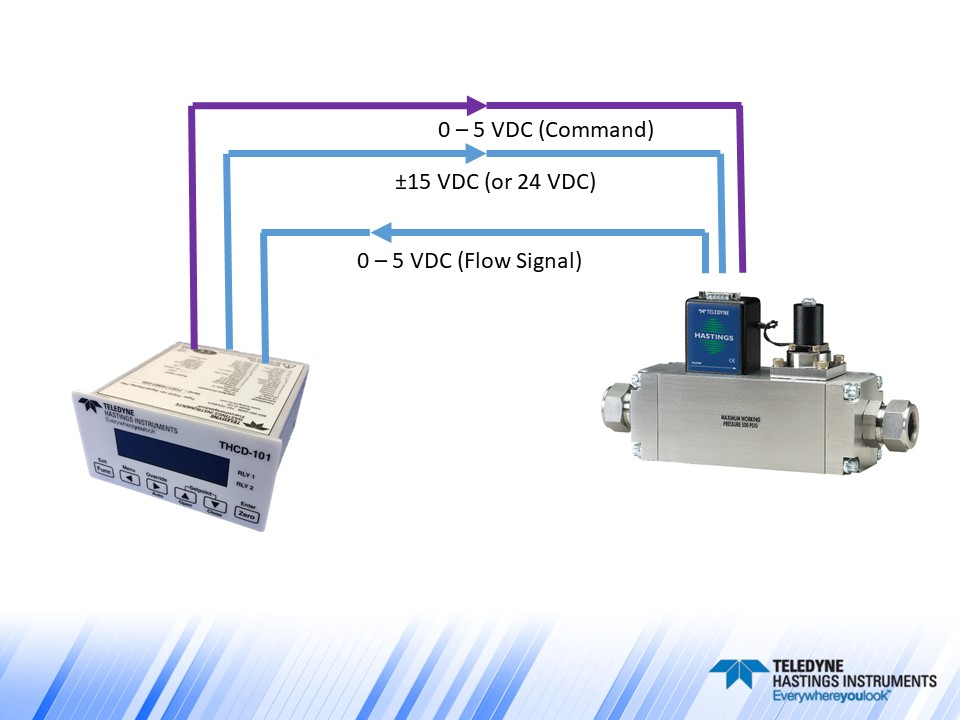Now, suppose we had an HFC-202 flow controller (200 Series) that was calibrated for 200 sccm of methane and we wanted to use it to control the flow of argon. What voltage level would we need on the command signal to have a flow rate of 100 sccm of argon? Let’s first determine the full-scale flow rate (5 VDC) when using argon:

Flow (Ar) = Flow (CH4)/GCF (CH4) * GCF (Ar) = (200 sccm / 0.77) * 1.401 = 363.9

So, a 5 VDC command signal will give us 363.9 sccm of argon. If we want 100 sccm, we would send:

Command Voltage = 100 sccm (5 VDC / 363.9 sccm) = 1.374 VDC.

Now, one important note about using flow controllers in different gases. Just because we can apply GCFs does not mean that a flow controller’s valve will work properly when switching from one gas to another. As an extreme example, a flow controller valve that has an orifice sized to handle hydrogen will have a hard time handling significant flows of large polyatomic molecules like C2H6.

Teledyne flow products are easy to install and use. And our application engineers are standing by to help. We can be reached by email (hastings_instruments@teledyne.com), by phone 757-723-6531, or via LiveChat on our website www.teledyne-hi.com or by clicking the contact us button below.Teledyne Hastings is proud to offer our Mass Flow Converter app. We have created a version for iPhone, iPad, and Droid. We have also created a web-based version that you can find at www.massflowconverter.com

In this blog article, we will discuss the motivation to build the app, how it works, and how it can be used.

The first question you might be asking is: Why do we need an app to convert from one set of mass flow units to another? For instance, if you want to convert from inches to centimeters, you would just multiply by 2.54. But, converting between mass flow units is not always that straight forward. So we have developed a tool that makes it easy.

We are going to look at some examples, but first let’s review what we mean by mass flow. When we think about mass flow, it can be helpful to think in terms of the flow of individual molecules. So while flow meters are often specified by units like sccm (standard cubic centimeters per minute) or scfm (standard cubic feet per minute), the mass flow rate is ultimately about the number of molecules (n) moving through a given cross sectional area per unit time (see figure below).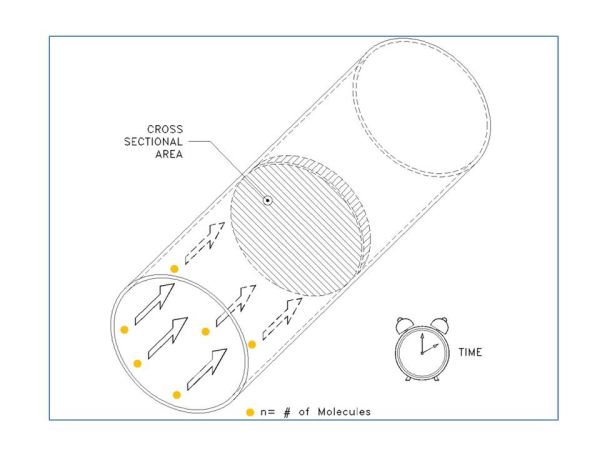So as our first example, let’s take a look at the conversion of 10,000 sccm (10,000 cm3/min) to a molecular flow rate. First, we need to ask, “How many molecules are in 10,000 sccm?” In the figure below, we show a container that is 10,000 cm3 in volume. Now, before we can calculate the number of gas molecules in a volume, we must know the pressure and temperature of the gas. We can use the ideal gas law:

n = (P * V) / R*T where n is the number of molecules, P is the pressure, V is the Volume, R is the Universal Gas Constant and T is the Temperature.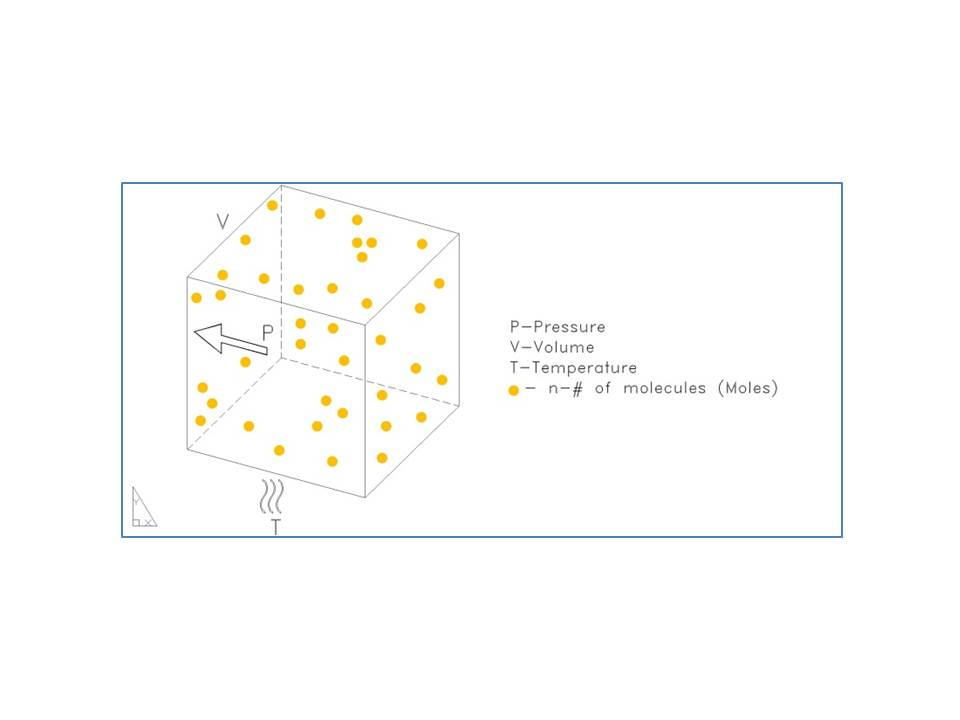Now we need to select some given pressure and temperature so that we can calculate the number of molecules – these are called the reference conditions or the STP (Standard Temperature and Pressure). In many cases, 0°C and 760 Torr are used for the STP. But this is not always the case. So it is always very important to specify the reference conditions (STP) any time you use a standardized mass flow unit like sccm, slm, scfh, etc (any mass flow unit that starts with “s” is going to need the reference conditions or STP specified). In our example, we are going to use STP of 0°C & 760 Torr.

OK, so here we go:     n = (1 atm) * (10,000 cc) / (82.053 cc * atm / K * mole) * (273 K)

Note that we have used a value of R in terms of pressure in Atmosphere (760 Torr = 1 atm), and Temperature in Kelvin (0°C = 273K).

n = 0.45 mole

In other words, a flow rate of 10,000 sccm (0C, 760 Torr) is the same as a molecular flow rate of 0.45 Mole / minute.

OK that is the hard way. It’s much easier to use the mass flow converter app. In the example shown above, we would dial sccm on the left and Mole/Min on the right. Then to select the reference conditions, we use the menu in the center. See Fig. 3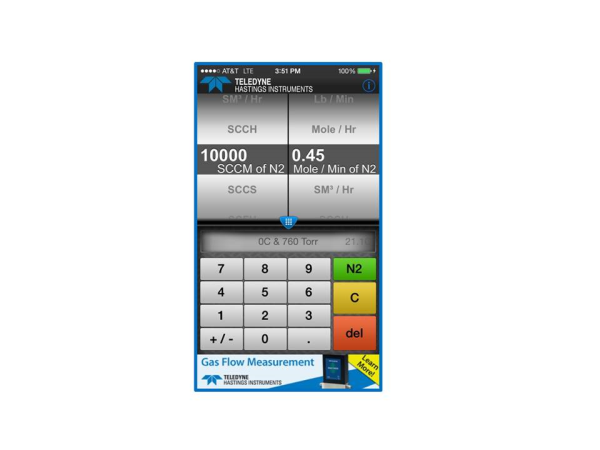If you are like me, you will start to play with the App. And soon you will notice that the user can change the gas using the pull down menu at the top. But notice that in the case of our first example (converting from standardized mass flow units to molecular flow units), that the gas selection has no effect on the conversion.  This is because the standardized flow units (e.g. sccm, slm, scfh, etc.) are actually molar flow units based on reference conditions (STP) and the ideal gas law.

So, why do we allow the user to select gas? In the case of units like gm/sec, Kg/hr, or lb / min, we are going to need to know the gas so that we can calculate the mass. Let’s take a look at the case of converting from slm to grams/second. We will use as our same example of 10,000 slm (0°C & 760 Torr) and we will use methane (CH4) as our gas.

We showed earlier that 10,000 sccm is a molecular flow rate of 0.45 Mole / Min.  And since 1 slm = 1000 sccm, it is easy to see that 10,000 slm = 450 Mol/min. And since we know that our unit of choice (gm/sec) is in terms of seconds, let’s go ahead and convert our time units now:

10,000 slm = (450 Mole / Min) * (1 Min / 60 sec) = 7.5 Mole/ sec.

Now we need to know how much mass there is in a Mole of methane. Google is very nice for getting this number – just type, “Molecular weight of methane” and here is the result: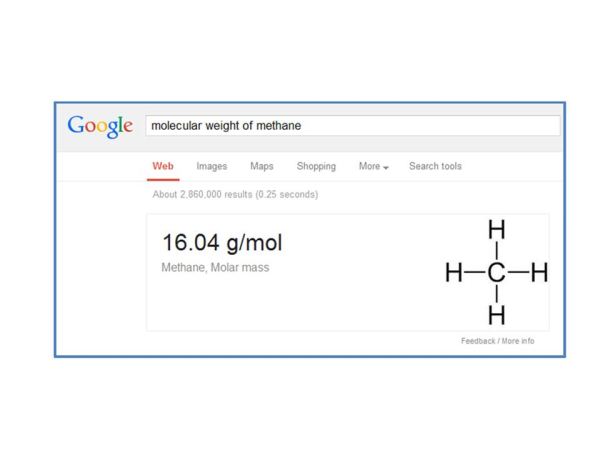By the way, Google will do this for almost all gases. So now we can finish our conversion and we get:

7.5 Mol / sec * (16.04 g/mol) = 120 g/sec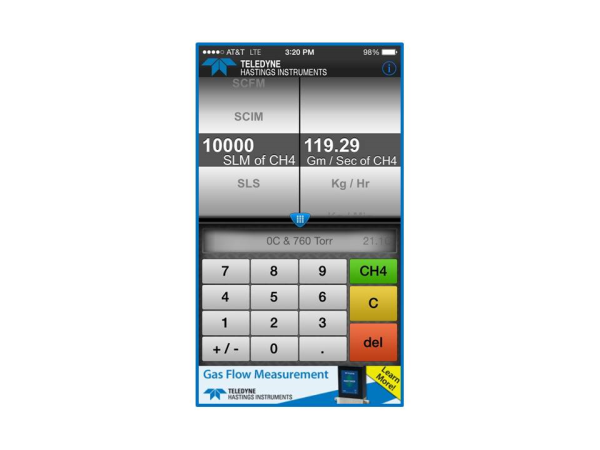The mass flow converter app and website www.massflowconverter.com takes all the work out of these conversions and we hope that you will find this tool helpful. If you have any questions about mass flow meters and controllers, Application Engineers at Teledyne Hastings are always happy to help.